Function Repository Resource:

# SerialRandomnessTest

Conduct an empirically derived test that assesses randomness using the frequencies of serial combinations of 0s and 1s

Contributed by: Emmy/Noah Blumenthal
noahb320@gmail.com
emmyb320@bu.edu
 ResourceFunction["SerialRandomnessTest"][sequence] conducts an empirically derived test using the frequencies of serial combinations of 0s and 1s in sequence and returns an associated p-value. ResourceFunction["SerialRandomnessTest"][sequence,"properties"] conducts an empirically derived test and returns the specified property.

## Details and Options

Properties include:
 "TestStatistic" returns the test statistic "PValue" returns the p-value associated with the test
The test statistic is generated by creating a chi-square-like statistic that compares the frequencies of subsequences of 0s and 1s of length two to the expected frequencies.
The test works only for sequences of 0s and 1s.
ResourceFunction["SerialRandomnessTest"] results are generally invalid for sequence lengths shorter than 100.

## Examples

### Basic Examples (3)

Generate a sequence of random integers:

 In:=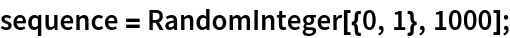Visualize the sequence:

 In:=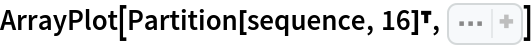Out=Apply a runs-based test:

 In:=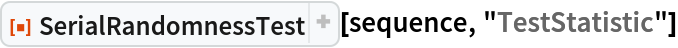Out=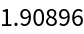In:=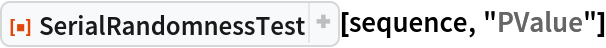Out=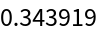### Applications (2)

Generate a sequence of random integers:

 In:=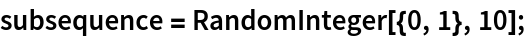In:=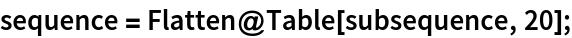Visualize the sequence:

 In:=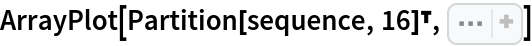Out=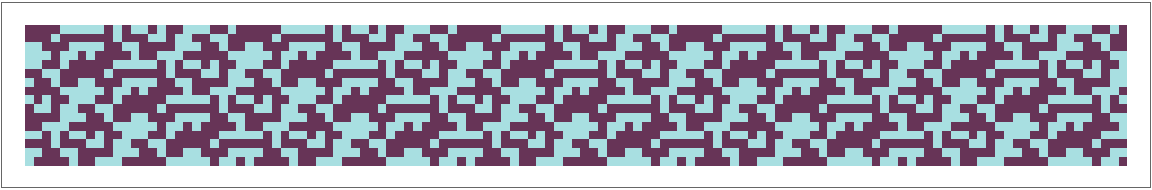Attempt to reject a non-random sequence:

 In:=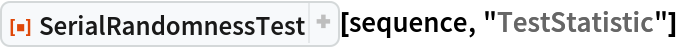Out=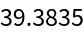In:=Out=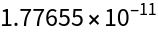Test the randomness of rule 30:

 In:=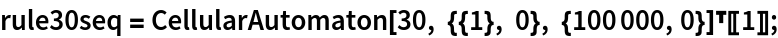In:=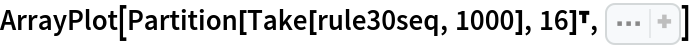Out=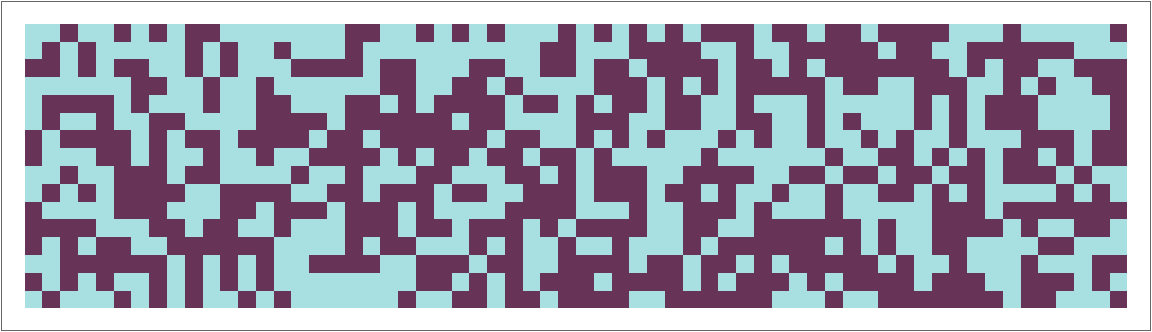In:=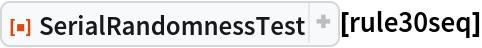Out=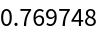### Possible Issues (1)

SerialRandomnessTest requires sequences of length 100 or more:

 In:=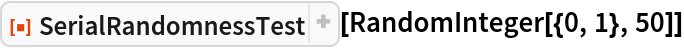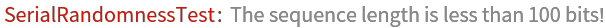### Neat Examples (1)

Visualize the sampling distribution of the test statistic:

 In:=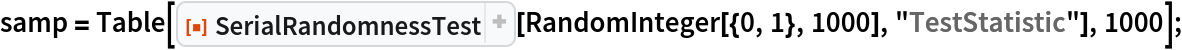In:=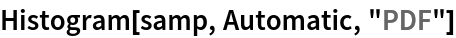Out=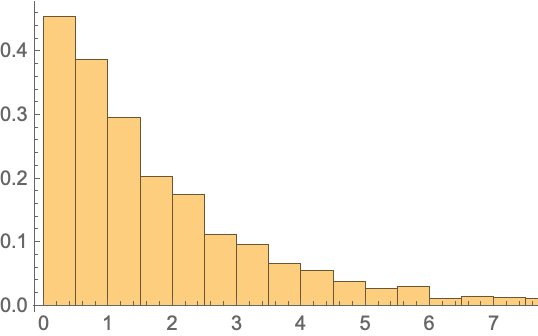In:=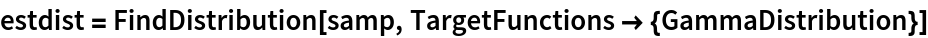Out=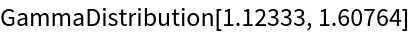In:=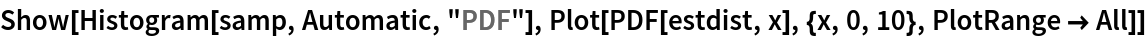Out=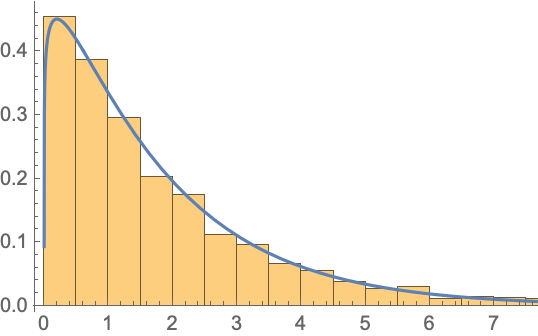Emmy Blumenthal

## Version History

• 1.0.0 – 08 July 2019